Courses

# Past Year Questions: Network Models Mechanical Engineering Notes | EduRev

## Mechanical Engineering : Past Year Questions: Network Models Mechanical Engineering Notes | EduRev

The document Past Year Questions: Network Models Mechanical Engineering Notes | EduRev is a part of the Mechanical Engineering Course Manufacturing and Industrial Engineering.
All you need of Mechanical Engineering at this link: Mechanical Engineering

Question 1:Consider the given project network, where numbers along various activities represent normal time. The free float on activity 4-6 and the project duration, respectively, are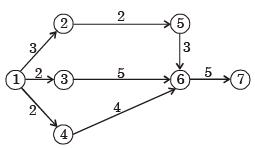Question 2:If duration of activity f is changed to 10 days, then the critical path for the project is



Question 3: The critical path for the project is



Question 4:The  project activities, precedence relationships and durations are described in the table. The critical path of the project is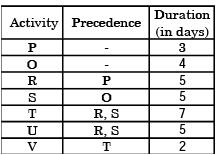Question 5: For the network below, the objective is to find the length of the shortest path from node P to node G. Let dij be the length of directed arc from node i to node j.Let sj, be the length of the shortest path from P to node j. Which of the following equations can be used to find SG?



Question 6:A project has six activities (A t o F ) w it h respective activity durations 7,5,6,6,8,4 days.The network has three path A-B, C-D and EF. All the activities can be crashed with the same crash cost per day.The number of activities that need to be crashed to reduce the project duration by 1 day is



Question 7:An assembly activity is represented on an Operation Process Chart by the symbol



Question 8: A project consists of activities A to M shown in the net in the following figure with the duration of the activities marked in daysThe project can be completed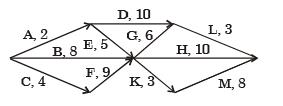Question 9:The symbol used for Transport in work study is



Question 10:In the construction of networks , dummy activities are introduced in order to



Question 11:In PERT chart, the activity time distribution is



Question 12:The standard deviation of the critical path is



Question 13:Consider the following PERT network: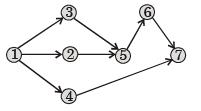The optimistic time, most likely time and pessimistic time of all the activities are given in the table below:The critical path duration of the network (in days) is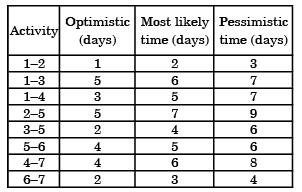Question 14:The expected time (te) of a PERT activity in terms of optimistic time (t0), pessimistic time (tp) and most likely time (tl) is given by



Question 15:The standard deviation of the critical path of the project is



Question 16:Consider a PERT network for a project involving six tasks (a to f)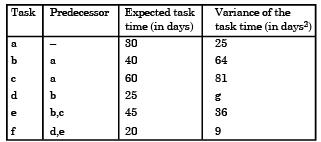The expected completion time of the project is



Question 17: In PERT analysis a critical activity has



Question 18:A dummy activity is used in PERT network to describe

Question 19: In PERT, the distribution of activity times is assumed to be



Offer running on EduRev: Apply code STAYHOME200 to get INR 200 off on our premium plan EduRev Infinity!

## Manufacturing and Industrial Engineering

91 videos|52 docs|59 tests

,

,

,

,

,

,

,

,

,

,

,

,

,

,

,

,

,

,

,

,

,

;